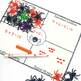# Multiplication with Manipulatives- Halloween Spider MathSubject
Resource Type
File Type

PDF

(9 MB|51 pages)
Product Rating
Standards
• Product Description
• StandardsNEW

Introduce students to the concept of multiplication with this hands-on unit. This spider-themed unit is perfect for fall, October, or Halloween. Use spider rings as your math manipulatives for great student engagement!

This beginning multiplication unit covers making arrays, multiplication as repeated addition, and equal groups in addition to the following properties of multiplication: zero, identity, and commutative. Because this is beginning multiplication, the associative and distributive properties are not directly covered. The spider array craftivity is a fun bulletin board or hallway display, perfect for showcasing your students' multiplication learning. Several centers and games are included as well.

WHAT'S INCLUDED:

• Directions for introducing multiplication with a Spider Manipulatives Hands– on Chart for products to 12
• Using manipulatives to understand multiplication & flip book
• Multiplication vocabulary posters
• Spider Craftivity
• Worksheets
• Games/Centers & Recording Sheets
• Factors cards
• Products cards

Please note: The preview does not include a preview of every page included. The centers are not shown in detail.

YOU MAY ALSO BE INTERESTED IN:

CLICK HERE to follow me and be notified of future products as soon as they are posted.

_______________________________________________________________

You may not redistribute, edit, sell, or otherwise post this product on the internet. You may, however, post a link for others to purchase themselves.

Fluently multiply and divide within 100, using strategies such as the relationship between multiplication and division (e.g., knowing that 8 × 5 = 40, one knows 40 ÷ 5 = 8) or properties of operations. By the end of Grade 3, know from memory all products of two one-digit numbers.
Apply properties of operations as strategies to multiply and divide. Examples: If 6 × 4 = 24 is known, then 4 × 6 = 24 is also known. (Commutative property of multiplication.) 3 × 5 × 2 can be found by 3 × 5 = 15, then 15 × 2 = 30, or by 5 × 2 = 10, then 3 × 10 = 30. (Associative property of multiplication.) Knowing that 8 × 5 = 40 and 8 × 2 = 16, one can find 8 × 7 as 8 × (5 + 2) = (8 × 5) + (8 × 2) = 40 + 16 = 56. (Distributive property.)
Determine the unknown whole number in a multiplication or division equation relating three whole numbers. For example, determine the unknown number that makes the equation true in each of the equations 8 × ? = 48, 5 = __ ÷ 3, 6 × 6 = ?.
Use multiplication and division within 100 to solve word problems in situations involving equal groups, arrays, and measurement quantities, e.g., by using drawings and equations with a symbol for the unknown number to represent the problem.
Interpret whole-number quotients of whole numbers, e.g., interpret 56 ÷ 8 as the number of objects in each share when 56 objects are partitioned equally into 8 shares, or as a number of shares when 56 objects are partitioned into equal shares of 8 objects each. For example, describe a context in which a number of shares or a number of groups can be expressed as 56 ÷ 8.
Total Pages
51 pages
N/A
Teaching Duration
N/A
Report this Resource to TpT
Reported resources will be reviewed by our team. Report this resource to let us know if this resource violates TpT’s content guidelines.